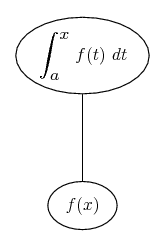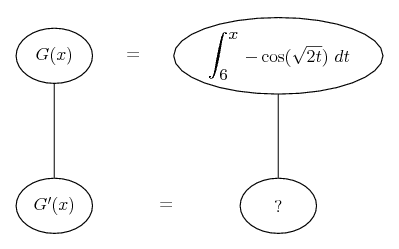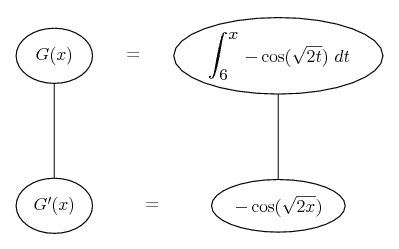# Thread: Fundamental Theorem of Calculus to find the derivative of the function

1. ## Fundamental Theorem of Calculus to find the derivative of the function

2. Firstly,

$\displaystyle G(x) = \int_x^6 \cos(\sqrt{2t}) dt = - \int_6^x \cos(\sqrt{2t}) dt$

The FTC says it then follows that $\displaystyle G'(x) = -\cos(\sqrt{2x})$

Just in case a picture helps...... and in this case...... where straight continuous lines differentiate downwards (integrate up) with respect to x.

Spoiler:__________________________________________

Don't integrate - balloontegrate!

Balloon Calculus: Gallery

Balloon Calculus Drawing with LaTeX and Asymptote!

#### Search Tags

calculus, calculus theorem, derivative, function, fundamental, integral, theorem